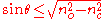xGuided rayEncyclopedia
A guided ray is a ray
Ray (optics)
In optics, a ray is an idealized narrow beam of light. Rays are used to model the propagation of light through an optical system, by dividing the real light field up into discrete rays that can be computationally propagated through the system by the techniques of ray tracing. This allows even very...

of light in a multi-mode optical fiber
Multi-mode optical fiber
Multi-mode optical fiber is a type of optical fiber mostly used for communication over short distances, such as within a building or on a campus...

, which is confined by the core. For step index fiber
Step-index profile
For an optical fiber, a step-index profile is a refractive index profile characterized by a uniform refractive index within the core and a sharp decrease in refractive index at the core-cladding interface so that the cladding is of a lower refractive index. The step-index profile corresponds to a...

, light entering the fiber will be guided if it falls within the acceptance cone of the fiber, that is if it makes an angle with the fiber axis that is less than the acceptance angle,,

where
θ is the angle the ray makes with the fiber axis, before entering the fiber,
no is the refractive index along the central axis of the fiber, and
nc is the refractive index of the cladding.

This result can be derived from Snell's law
Snell's law
In optics and physics, Snell's law is a formula used to describe the relationship between the angles of incidence and refraction, when referring to light or other waves passing through a boundary between two different isotropic media, such as water and glass...

by considering the critical angle
Critical angle
Critical angle can refer to:*Critical angle the angle of incidence above which total internal reflection occurs*Critical angle of attack, in aerodynamics; the angle of attack which produces the maximum lift coefficient...

.

Rays that fall within this angular range are reflected from the core-cladding boundary by total internal reflection
Total internal reflection
Total internal reflection is an optical phenomenon that happens when a ray of light strikes a medium boundary at an angle larger than a particular critical angle with respect to the normal to the surface. If the refractive index is lower on the other side of the boundary and the incident angle is...

, and so are confined by the core. The confinement of light by the fiber can also be described in terms of bound modes or guided modes. This treatment is necessary when considering singlemode fiber, since the ray model does not accurately describe the propagation of light in this type of fiber.